Space, shape and measurement: Use the Cartesian co-ordinate system to derive and apply equations

# Unit 1: Plotting points on the Cartesian plane

Dylan Busa### Unit outcomes: Unit 1: Plotting points on the Cartesian plane

By the end of this unit you will be able to:

• Use the Cartesian co-ordinate system to plot points, lines and polygons.
• Find co-ordinates from labelled points.

## What you should know

Before you start this unit, make sure you can:

• State the key features of the square, rectangle, hexagon, trapezium, and triangle. Refer to level 2 subject outcome 3.2 unit 1 if you need help with this.

## Introduction

Where in the world are you? How would you explain to someone else on the other side of the world where you are right now? You might say you are in your house or at college. But where is that? You might give the address of your house or college for the person to look up on the internet. But how do satellite navigation systems or Google Maps know where your address actually is? It all comes down to co-ordinates.

## Co-ordinates

Co-ordinates are a set of two numbers that describe your position exactly and consistently. These numbers are your longitude (how far East or West you are) and your latitude (how far North or South you are).

The Union Buildings in Pretoria, for example, have the following co-ordinates:
Latitude: $\scriptsize -25.740610122680664$
Longitude: $\scriptsize 28.21186637878418$

This means that they are $\scriptsize 28.21186637878418$ degrees East of (or to the right of) the Prime Meridian and $\scriptsize -25.740610122680664$ degrees South of (or below) the Equator (see Figure 1). The Prime Meridian is an imaginary line, like the Equator, but that runs from the North Pole to the South Pole dividing the Earth into East and West.

We can write these co-ordinates as an ordered pair.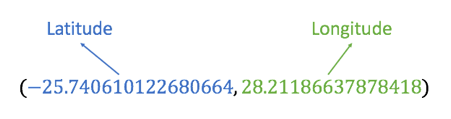We call this an ordered pair because there are two numbers (a pair) and they are always given in the same order (latitude then longitude).

Every time you have used a map or navigation system you have used this global positioning system. Such a system, which is defined by two perpendicular number lines, is called a Cartesian system in honour of the mathematician, Rene Descartes, who formalised its use in mathematics.

### Did you know?

The place on Earth with co-ordinates $\scriptsize (0,0)$ is called Null Island . There is a little weather buoy (see Figure 2) anchored in this location. You can learn more about Null Island on Wikipedia.

## The Cartesian plane

The Cartesian plane is a two-dimensional surface defined by two perpendicular number lines which we call axes. The horizontal axis (running from left to right) is the x-axis and the vertical axis (running from top to bottom) is the y-axis. Each axis extends forever in both directions this is why we draw them with arrows on the ends.

We call the point where the axes meet the origin.

We can describe the position of any point on the Cartesian plane with an ordered pair. The first number is the x-coordinate (how far left or right of the origin the point is) and the second number is the y-coordinate (how far up or down from the origin the point is).

Figure 4 shows the position of the points $\scriptsize \text{A}(2,4)$, $\scriptsize \text{B}(-3,1)$ and $\scriptsize \text{C}(4,-3)$ on the Cartesian plane. We can see that point $\scriptsize \text{A}$ is $\scriptsize 2$ units to the right of the origin and $\scriptsize 4$ units up from the origin. Point $\scriptsize \text{B}$ is $\scriptsize 3$ units to the left of the origin (it is a negative number) and $\scriptsize 1$ unit up from the origin. Point $\scriptsize \text{C}$ is $\scriptsize 4$ units to the right of the origin and $\scriptsize 3$ units below the origin (it is a negative number).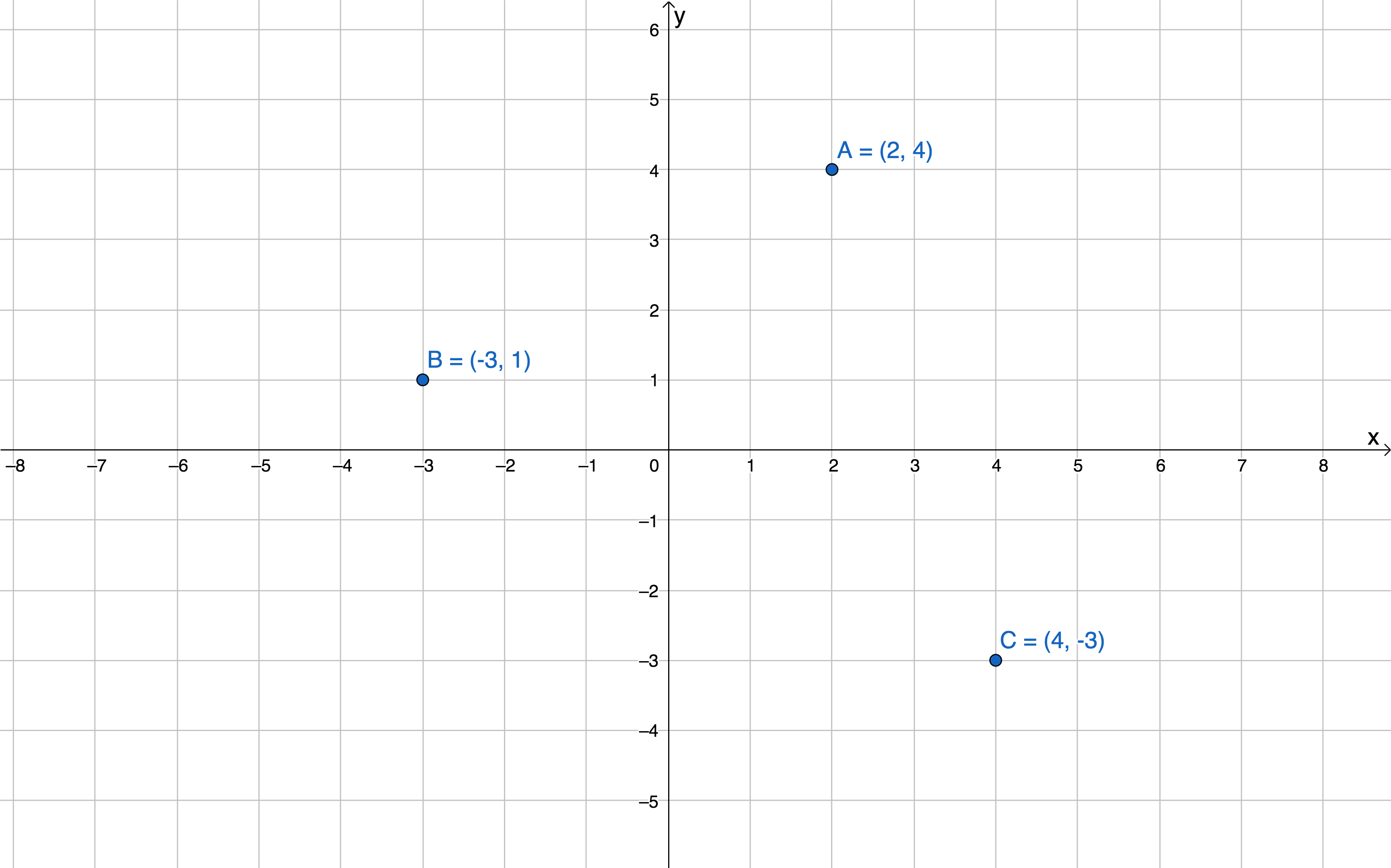Figure 4: The locations of points $\scriptsize \text{A}$, $\scriptsize \text{B}$ and $\scriptsize \text{C}$ on the Cartesian plane### Example 1.1

1. Determine the coordinates of points $\scriptsize \text{A}$, $\scriptsize \text{B}$ and $\scriptsize \text{C}$ on the Cartesian plane.2. Plot the following points on the same Cartesian plane.
1. $\scriptsize \text{P}(4,7)$
2. $\scriptsize \text{Q}(1,-7)$
3. $\scriptsize \text{R}(-3,-4.5)$
4. $\scriptsize \text{S}\left( {-3,0} \right)$

Solutions

1. Point $\scriptsize \text{A(2,3)}$:Point $\scriptsize \text{B(-3,5)}$: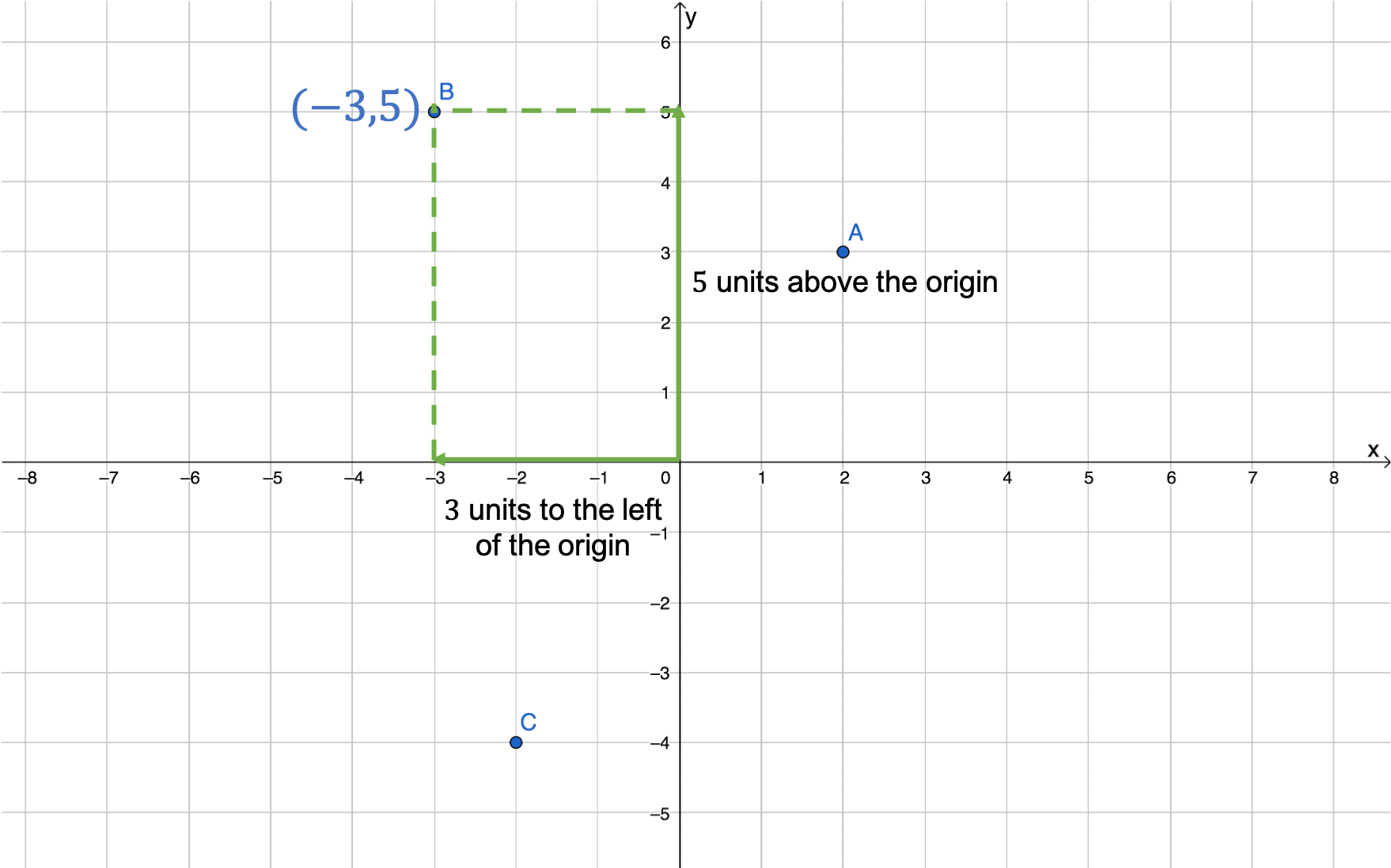Point $\scriptsize \text{C(-2,-4)}$: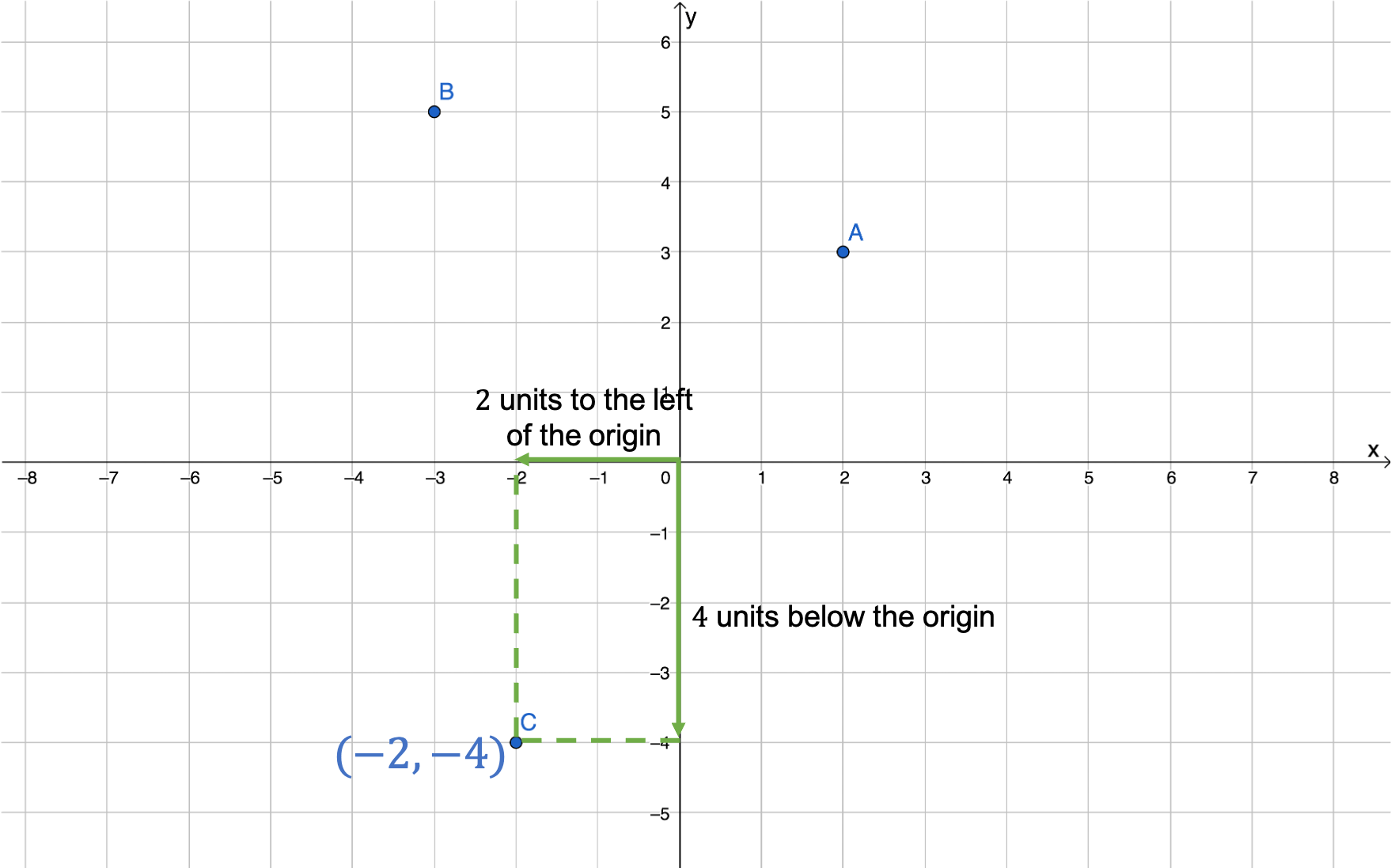2. Here are the points plotted on the same Cartesian plane. Notice how $\scriptsize \text{R}(-3,-4.5)$ is halfway between $\scriptsize -4$ and $\scriptsize -5$ on the y-axis. Notice how $\scriptsize \text{S}\left( {-3,0} \right)$ lies on the x-axis. This is because its y-coordinate is zero and so it is neither above nor below the origin.### Note

In Question 2 in Example 1.1, it was necessary for us to draw our own Cartesian plane. When you draw your own Cartesian plane, take note of the following properties.

• The axes must be perpendicular (at right angles) to each other.
• There must be an arrowhead at both ends of each axis.
• You must label the axes $\scriptsize x$ and $\scriptsize y$.
• You must label the origin $\scriptsize 0$.
• You must add a scale to each axis and label them with whole numbers.

You may have noticed that the two axes on the Cartesian plane divide the plane into four pieces. We call these quadrants and label them quadrant 1 (or quadrant I), quadrant 2 (or quadrant II), quadrant 3 (or quadrant III), and quadrant 4 (or quadrant IV) as shown in Figure 5.

• Coordinates in quadrant 1 have positive x-value and y-values.
• Coordinates in quadrant 2 have negative x-values and positive y-values.
• Coordinates in quadrant 3 have negative x-values and y-values.
• Coordinates in quadrant 4 have positive x-values and negative y-values.

### Note

If you would like to practise plotting points on the Cartesian plane try the interactive activities called Plot points in quadrant 1If you would like more practise finding the coordinates of points on the Cartesian plane try the interactive activity called Plotting and finding coordinates.### Exercise 1.1

1. Plot the following points on the same system of axes (this means on the same Cartesian plane).
1. $\scriptsize \text{H}(-2,1.5)$
2. $\scriptsize \text{I}(0,-4)$
3. $\scriptsize \text{J}(6,0)$
4. $\scriptsize \text{K}(-4,-5)$
5. $\scriptsize \text{L}(0,0)$
2. What are the coordinates of each of the points $\scriptsize \text{A}$ to $\scriptsize \text{F}$.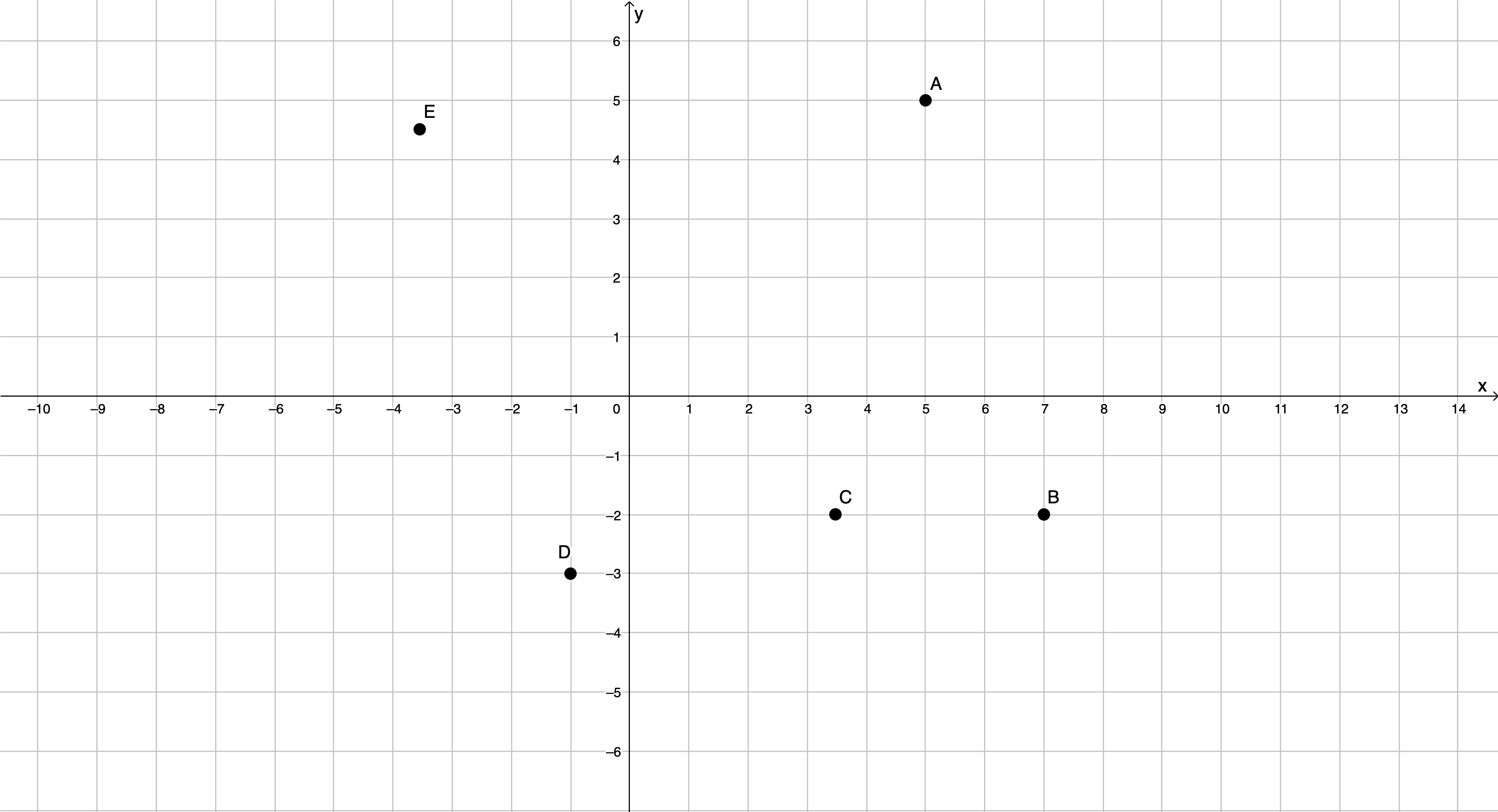3. In which quadrant will the following points be located?
1. $\scriptsize (6,7)$
2. $\scriptsize (-6,-7)$
3. $\scriptsize (-1,3)$
4. $\scriptsize (\pi ,-4)$

The full solutions are at the end of the unit.

### Note

If you have an Internet connection, watch these two videos for a summary of what we have learned so far.

Axis and Coordinates (Duration: 2.46)The Cartesian Plane (Duration: 7.44)## Lines and polygons on the Cartesian plane

In Subject outcome 3.2, Unit 1 you learnt about polygons. Do you recall that a polygon is any closed shape with straight sides? Figure 6 shows some examples of polygons. To revise more about polygons, refer back to that unit.

In the next activity we will create polygons on a Cartesian plane by plotting points and joining them with straight lines to form shapes.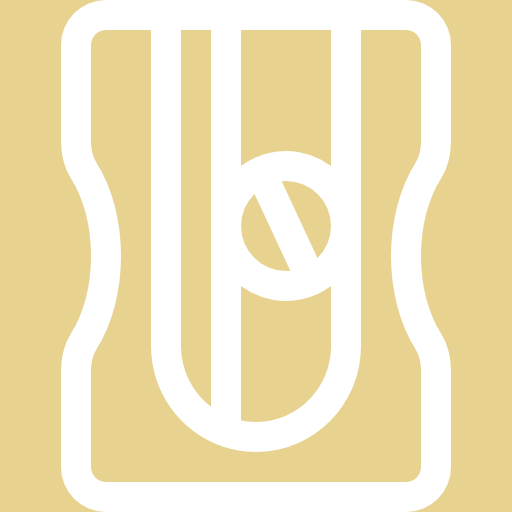### Activity 1.1: Join the dots

Time required: 20 minutes

What you need:

• a piece of paper
• coloured pens or pencils

What to do:

Draw your own Cartesian plane on a piece of paper. You need to make sure that the x-axis extends from $\scriptsize -3$ to $\scriptsize 13$ and that the y-axis extends from $\scriptsize -1$ to $\scriptsize 9$.

1. Plot the following points: $\scriptsize \text{O}(0,0)$, $\scriptsize \text{B}(0,5)$, $\scriptsize \text{C}(8,5)$, $\scriptsize \text{D}(8,0)$. Join these points in the following order: $\scriptsize \text{O,B,C,D}$. What shape does this make?
2. Plot the following points: $\scriptsize \text{E}(-2,5)$, $\scriptsize \text{F}(4,8)$, $\scriptsize \text{G}(10,5)$. Join these points in the following order: $\scriptsize \text{E,F,G}$. What shape does this make?
3. Plot the following points: $\scriptsize \text{H}(7,0)$, $\scriptsize \text{I}(7,3)$, $\scriptsize \text{J}(5,3)$, $\scriptsize \text{K}(5,0)$. Join these points in the following order: $\scriptsize \text{H,I,J,K}$. What shape does this make?
4. Plot the following points: $\scriptsize \text{L}(1,3)$, $\scriptsize \text{M}(3,3)$, $\scriptsize \text{N}(3,1)$, $\scriptsize \text{P}(5,0)$. Join these points in the following order: $\scriptsize \text{L,M,N,P}$. What shape does this make?
5. Plot the following points: $\scriptsize \text{Q}(2,1)$, $\scriptsize \text{R}(2,3)$. Join these points in the following order: $\scriptsize \text{Q,R}$. What shape does this make?
6. Plot the following points: $\scriptsize \text{S}(3,2)$, $\scriptsize \text{T}(1,2)$. Join these points in the following order: $\scriptsize \text{S,T}$. What shape does this make?
7. Plot the following points: $\scriptsize \text{U}(0,8)$, $\scriptsize \text{V}(1,8)$, $\scriptsize \text{W}(1,6.5)$, $\scriptsize \text{X}(0,6)$. Join these points in the following order: $\scriptsize \text{U,V,W,X}$. What shape does this make?
8. Colour $\scriptsize \text{UVWX}$ in brown. Colour $\scriptsize \text{LMNP}$ in blue. Make $\scriptsize \text{QR}$ and $\scriptsize \text{ST}$ black. Colour $\scriptsize \text{HIJK}$ green. Colour $\scriptsize \text{EFG}$ red. Colour the rest of $\scriptsize \text{OBCD}$ blue. What have you drawn?

What you found:

1. $\scriptsize \text{OBCD}$ is a rectangle.
2. $\scriptsize \text{EFG}$ is a triangle.
3. $\scriptsize \text{HIJK}$ is a rectangle.
4. $\scriptsize \text{LMNP}$ is a square.
5. $\scriptsize \text{QR}$ is a straight line segment.
6. $\scriptsize \text{ST}$ is a straight line segment.
7. $\scriptsize \text{UVWX}$ is a quadrilateral.
8. Here is the picture you should have drawn.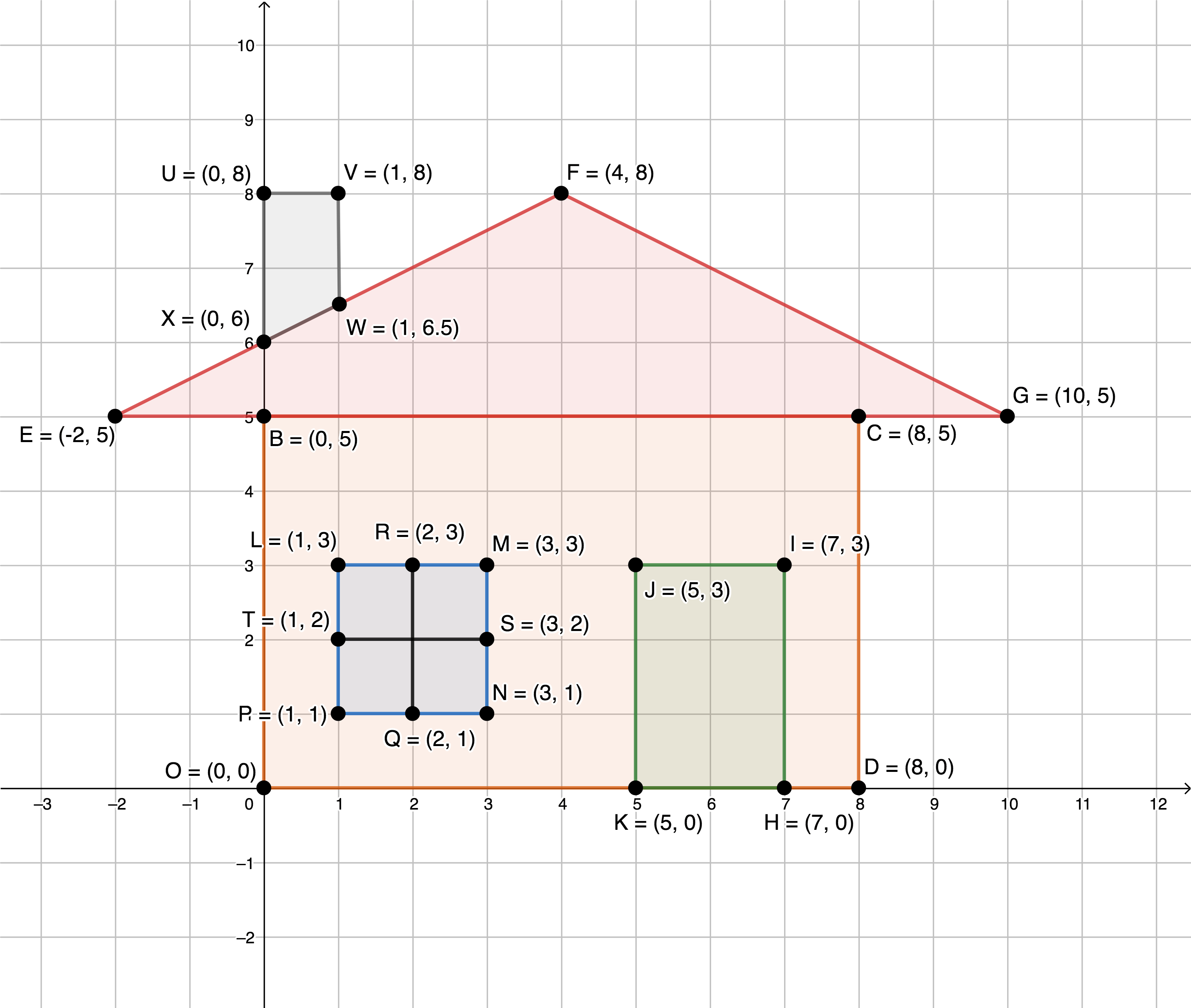From Activity 1.1, we can see that we can use sets of ordered pairs on the Cartesian plane to draw polygons and lines. In fact, we can draw any polygon we like. Remember a polygon is a closed shape with straight sides. The more sides there are, the more ordered pairs we need.

Have a look at $\scriptsize \text{OBCD}$ that you drew in Activity 1.1 again. Did the order in which we joined the points with straight lines matter? In order for us to draw the rectangle, we had to go from point $\scriptsize \text{O}$ to $\scriptsize \text{B}$ to $\scriptsize \text{C}$ and then to $\scriptsize \text{D}$. What other orders would result in the same rectangle? $\scriptsize \text{BCDO}$, $\scriptsize \text{CDOB}$ and $\scriptsize \text{DOBC}$ would all work as well.

However, can you see that if we went from point $\scriptsize \text{O}$ to $\scriptsize \text{C}$ to $\scriptsize \text{B}$ to $\scriptsize \text{D}$ we would not have ended up with the rectangle.

So, the order in which we join the points matters and this order is represented in the name we give to the polygon, for example, $\scriptsize \text{OBCD}$.

### Note

When you draw polygons on the Cartesian plane, the order of the letters for naming the figure is important. It indicates the order in which the points must be joined.For example here we have: $\scriptsize \text{A}$ to $\scriptsize \text{B}$, $\scriptsize \text{B}$ to $\scriptsize \text{C}$, $\scriptsize \text{C}$ to $\scriptsize \text{D}$, $\scriptsize \text{D}$ to $\scriptsize \text{E}$, $\scriptsize \text{E}$ to $\scriptsize \text{F}$, and $\scriptsize \text{F}$ back to $\scriptsize \text{A}$. The above quadrilateral can also be referred to as quadrilateral $\scriptsize \text{ABCDEF}$ or $\scriptsize \text{CDEFAB}$ or $\scriptsize \text{DEFABC}$.

However, it is conventional to write the letters in alphabetical order.### Exercise 1.2

1. What shapes do the following points create when you join them in the order in which they are given below? You will need to draw each shape on a Cartesian plane to check your answers.
1. $\scriptsize \text{A}(-4,2)$, $\scriptsize \text{B}(-4,-4)$, $\scriptsize \text{C}(6,-4)$
2. $\scriptsize \text{D}(-4,3)$, $\scriptsize \text{E}(3,3)$, $\scriptsize \text{F}(3,-1)$, $\scriptsize \text{G}(-4,-1)$
3. $\scriptsize \text{H}(0,-2)$, $\scriptsize \text{I}(2,0)$, $\scriptsize \text{J}(0,2)$, $\scriptsize \text{K}(-2,0)$
2. Work out the coordinates of the point $\scriptsize \text{N}$ so that $\scriptsize \text{LMNO}$ is a square if $\scriptsize \text{L}(-3,2)$, $\scriptsize \text{M}(-1,5)$ and $\scriptsize \text{O}$ is the origin $\scriptsize (0,0)$?

The full solutions are at the end of the unit.

## Summary

In this unit you have learnt the following:

• What the Cartesian plane is and what ordered pairs are.
• How to plot points on the Cartesian plane.
• How to identify the coordinates of points on the Cartesian plane.
• How to draw lines and polygons on the Cartesian plane.

# Unit 1: Assessment

#### Suggested time to complete: 15 minutes

1. What are the coordinates of points $\scriptsize \text{A}$, $\scriptsize \text{B}$ and $\scriptsize \text{C}$?2. Which point has coordinates $\scriptsize (0,-2)$?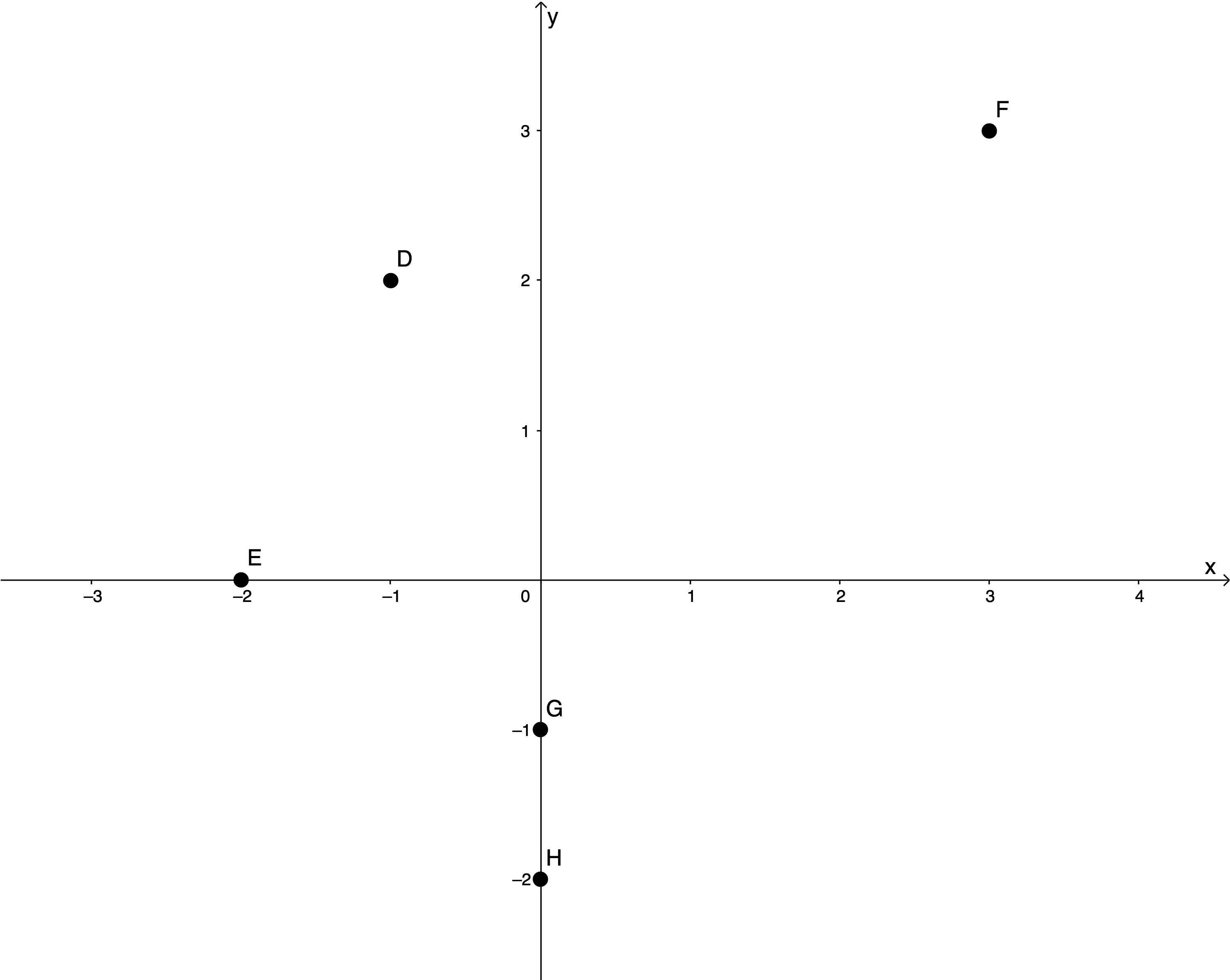3. $\scriptsize \text{ABCD}$ is a rectangle on the Cartesian plane. What are the coordinates of $\scriptsize \text{D}$ if $\scriptsize \text{A}(-2,3)$, $\scriptsize \text{B}(5,3)$ and $\scriptsize \text{C}(5,-5)$?

Bonus question:

$\scriptsize \text{IJ}$ and $\scriptsize \text{KL}$ are line segments that are parallel and of equal length. If $\scriptsize \text{I}(2,2)$, $\scriptsize \text{J}(0,-3)$, and $\scriptsize \text{L}(-1,-1)$, what are the two possible sets of coordinates for $\scriptsize \text{K}$?

The full solutions are at the end of the unit.

# Unit 1: Solutions

### Exercise 1.1

1. .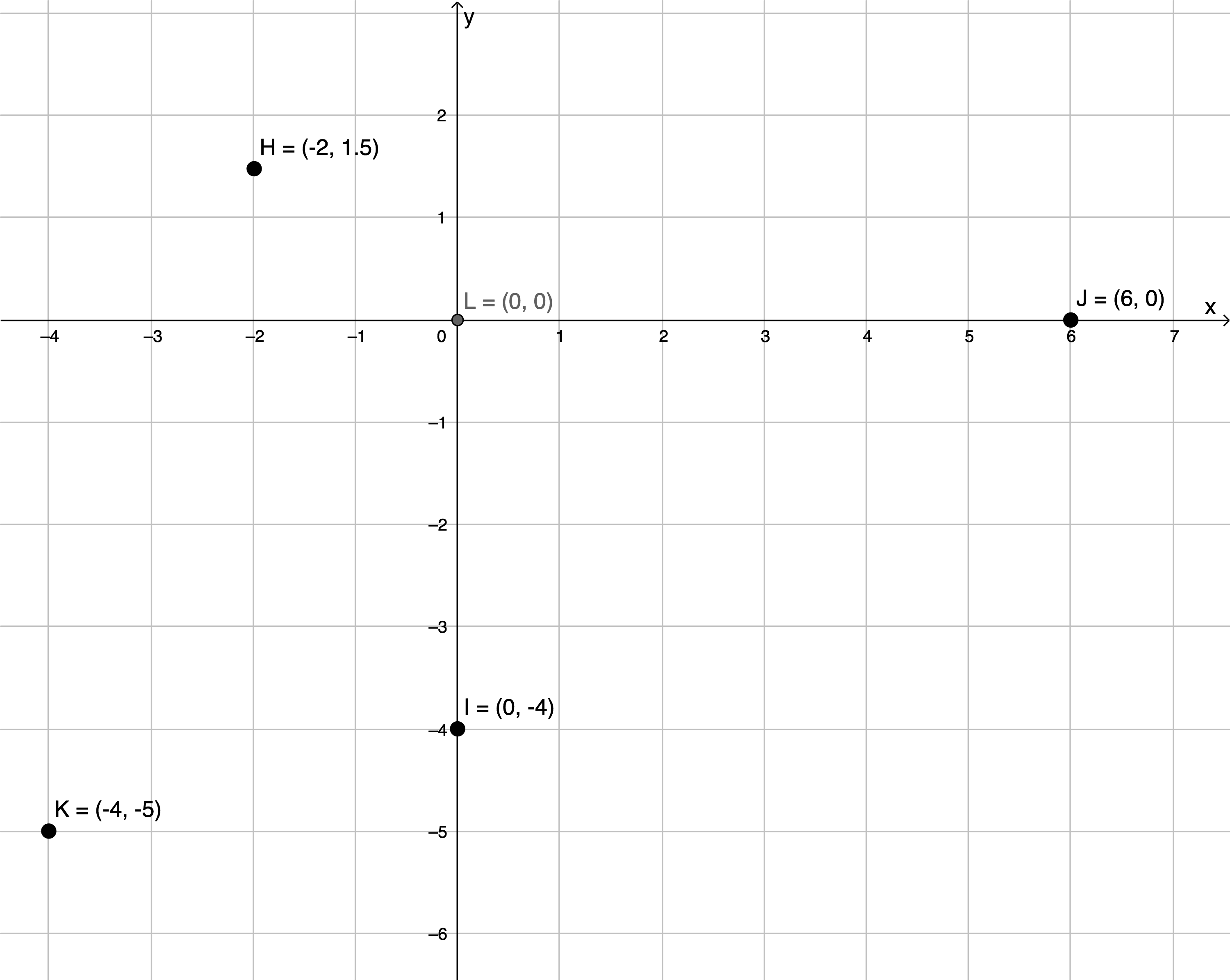2. .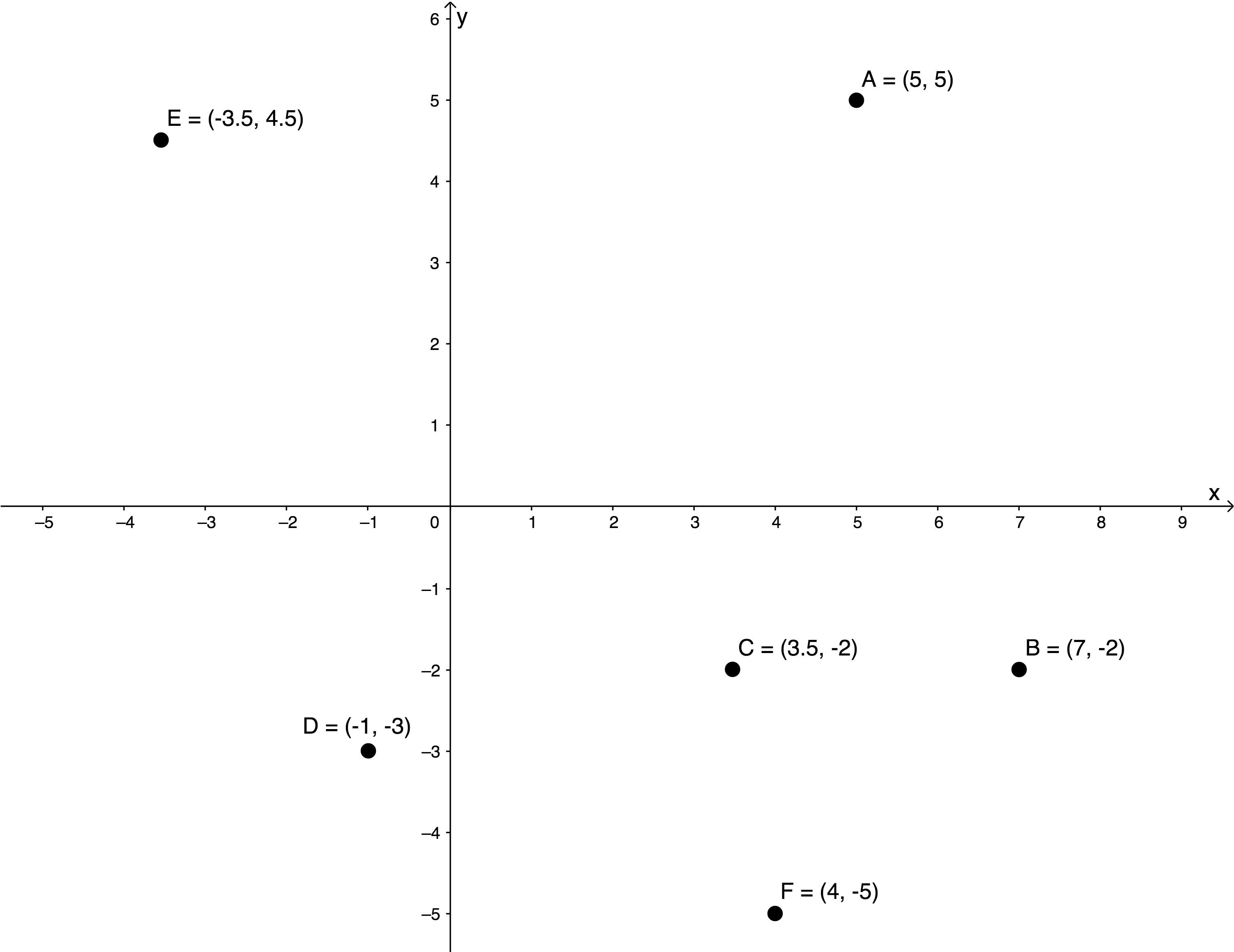3. .
1. $\scriptsize (6,7)$ is in quadrant 1.
2. $\scriptsize (-6,-7)$ is in quadrant 3.
3. $\scriptsize (-1,3)$ is in quadrant 2.
4. $\scriptsize (\pi ,-4)$ is in quadrant 4.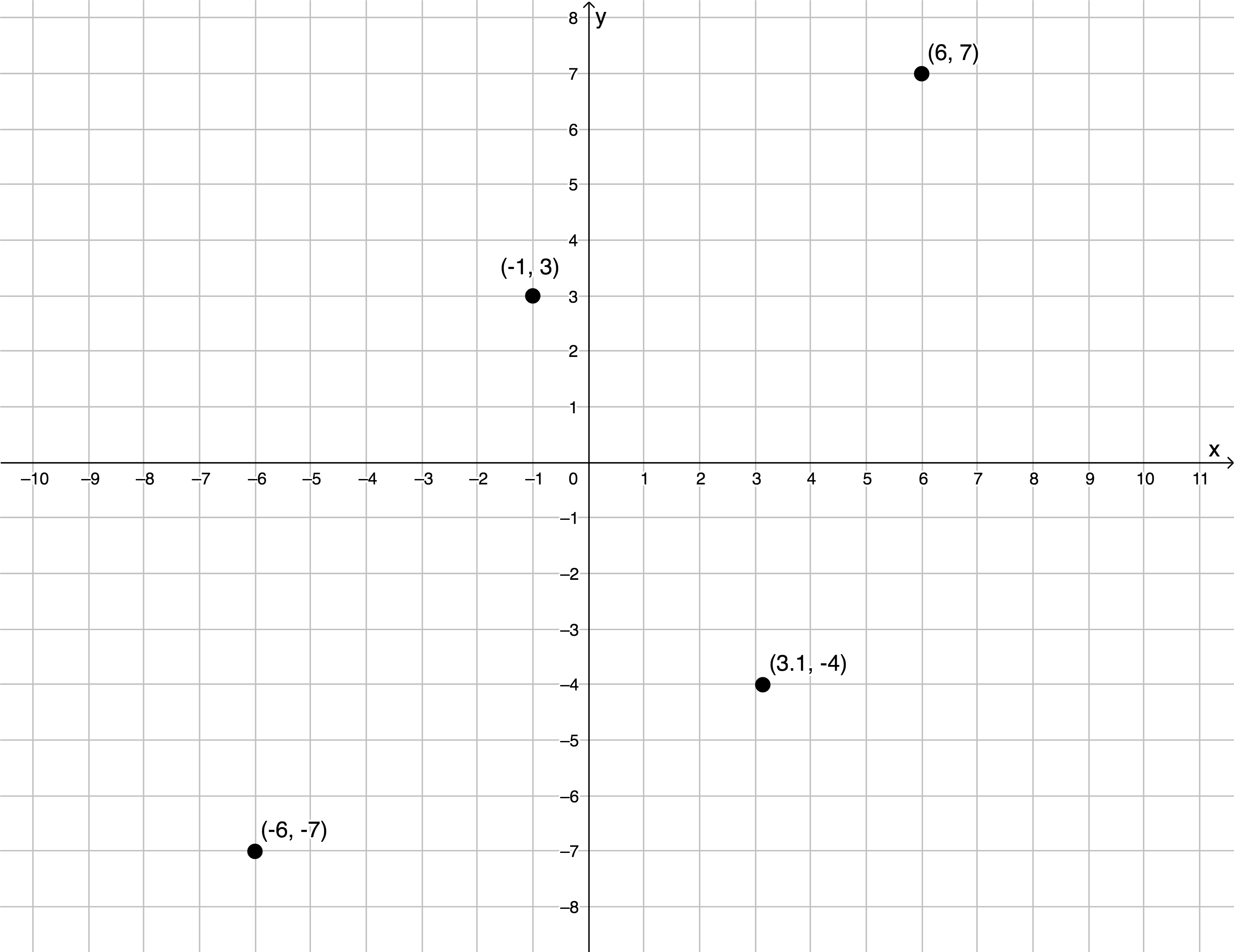Back to Exercise 1.1

### Exercise 1.2

1. .
1. $\scriptsize \text{ABC}$ is a right-angled triangle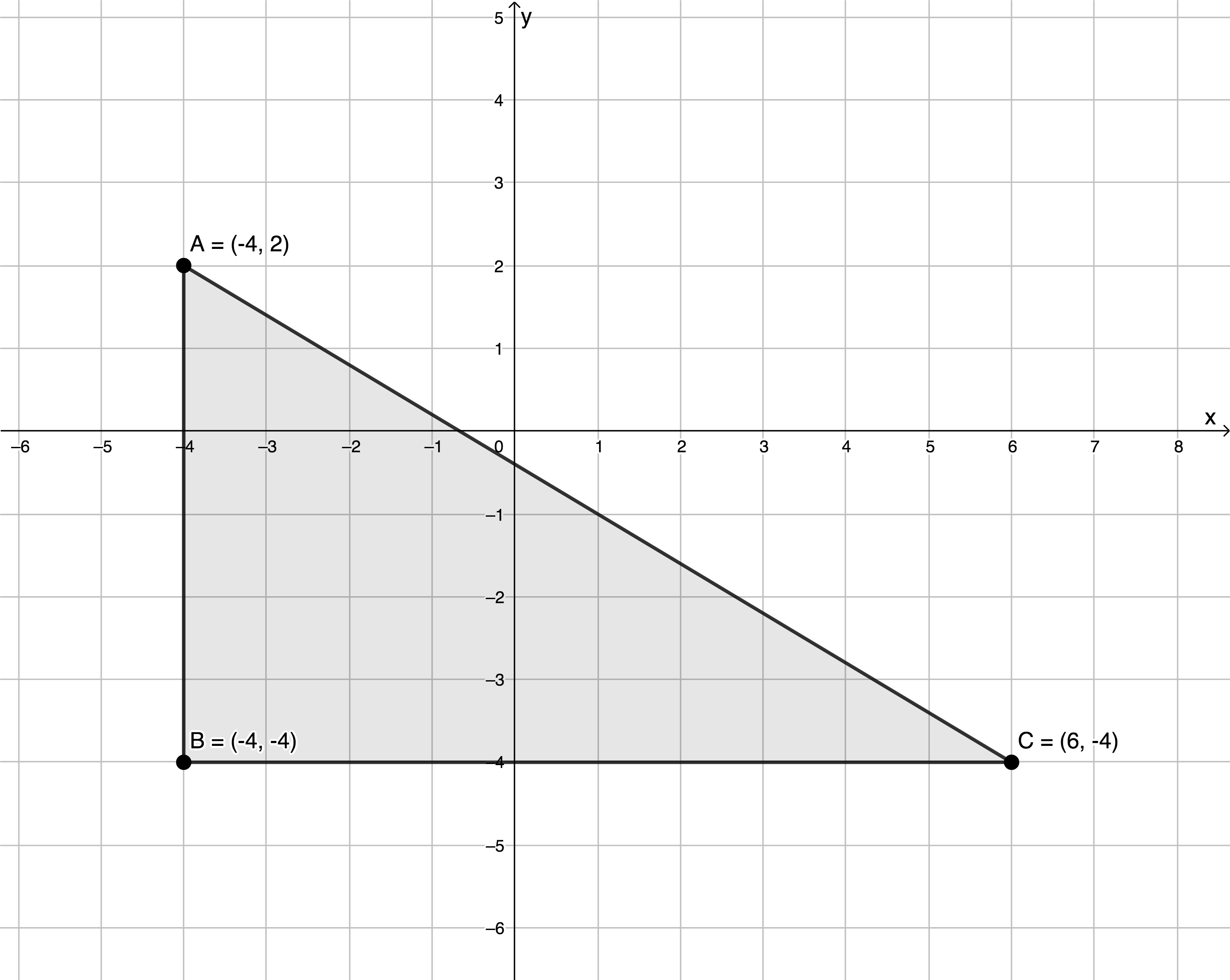2. $\scriptsize \text{DEFG}$ is a rectangle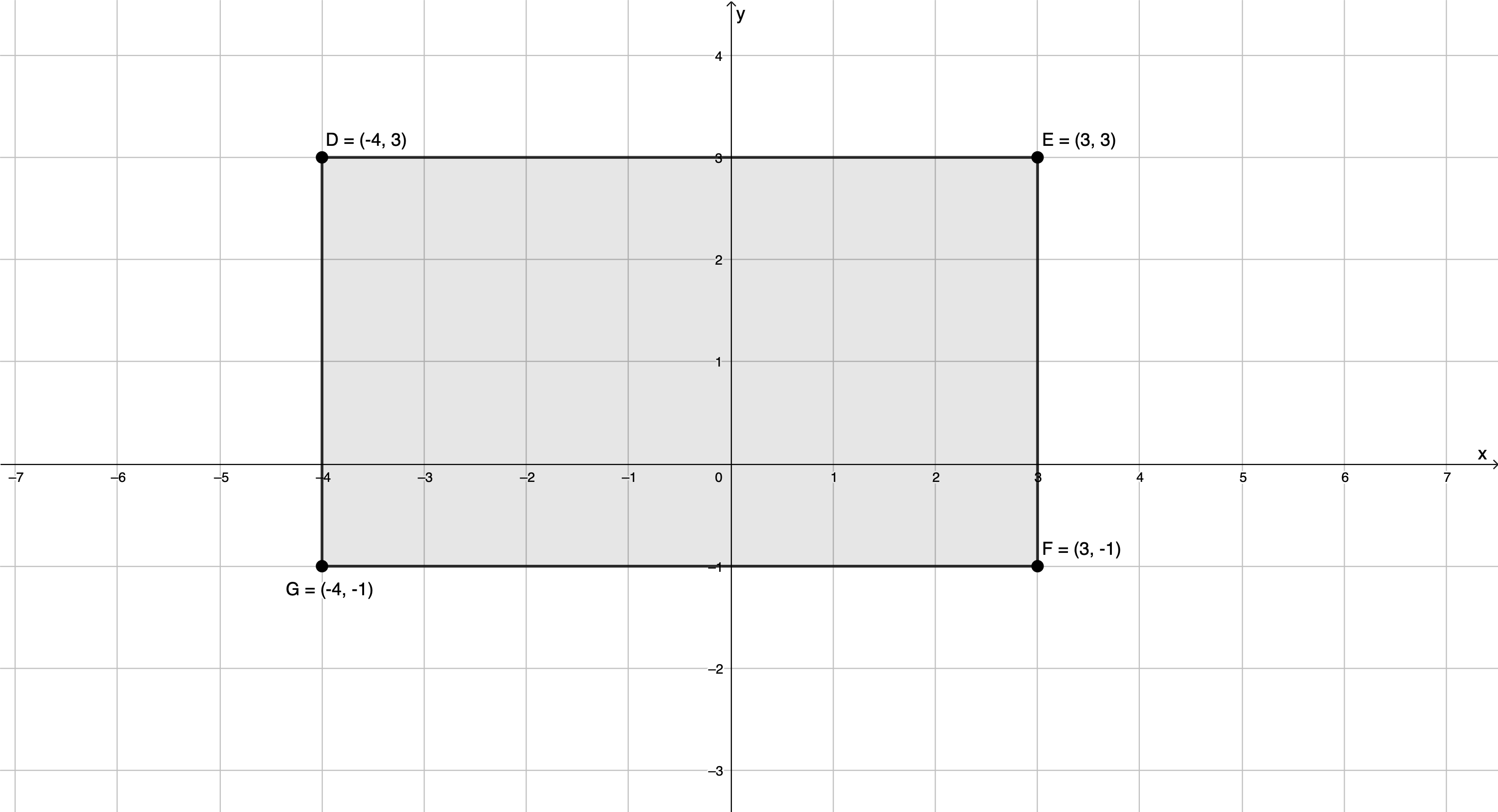3. $\scriptsize \text{HIJK}$ is a square2. $\scriptsize \text{N}$ must be the point $\scriptsize (2,3)$ in order for $\scriptsize \text{LMNO}$ to be a square.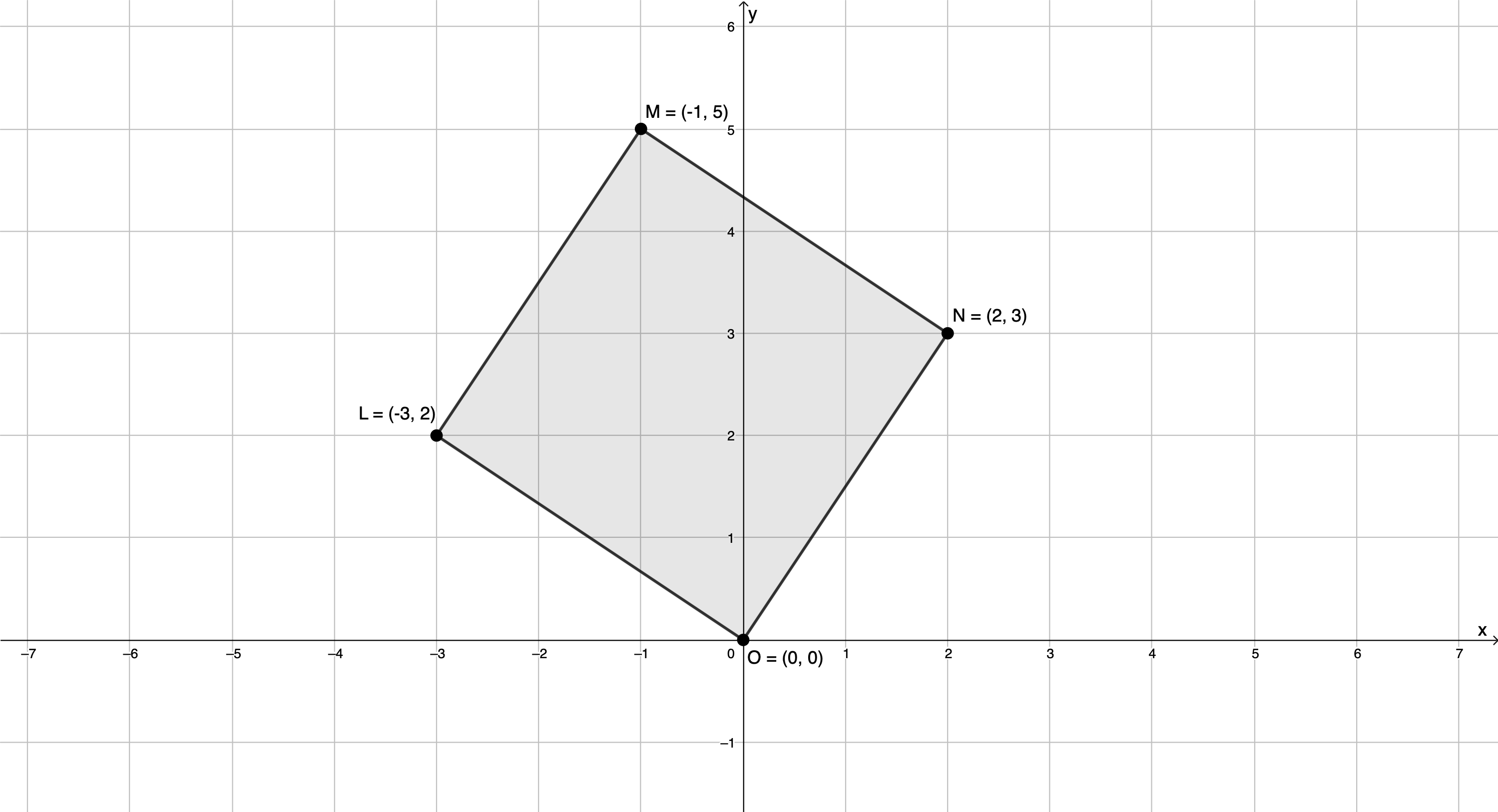Back to Exercise 1.2

### Unit 1: Assessment

1. $\scriptsize \text{A}(-4,0)$, $\scriptsize \text{B}(1,-2)$, $\scriptsize \text{C}(3.5,2)$
2. $\scriptsize \text{H}(0,-2)$
3. If we plot points $\scriptsize \text{A}$, $\scriptsize \text{B}$ and $\scriptsize \text{C}$, we can see that $\scriptsize \text{D}$ must be the point $\scriptsize (-2,-5)$.Bonus question:
First, we need to plot the information we have been given.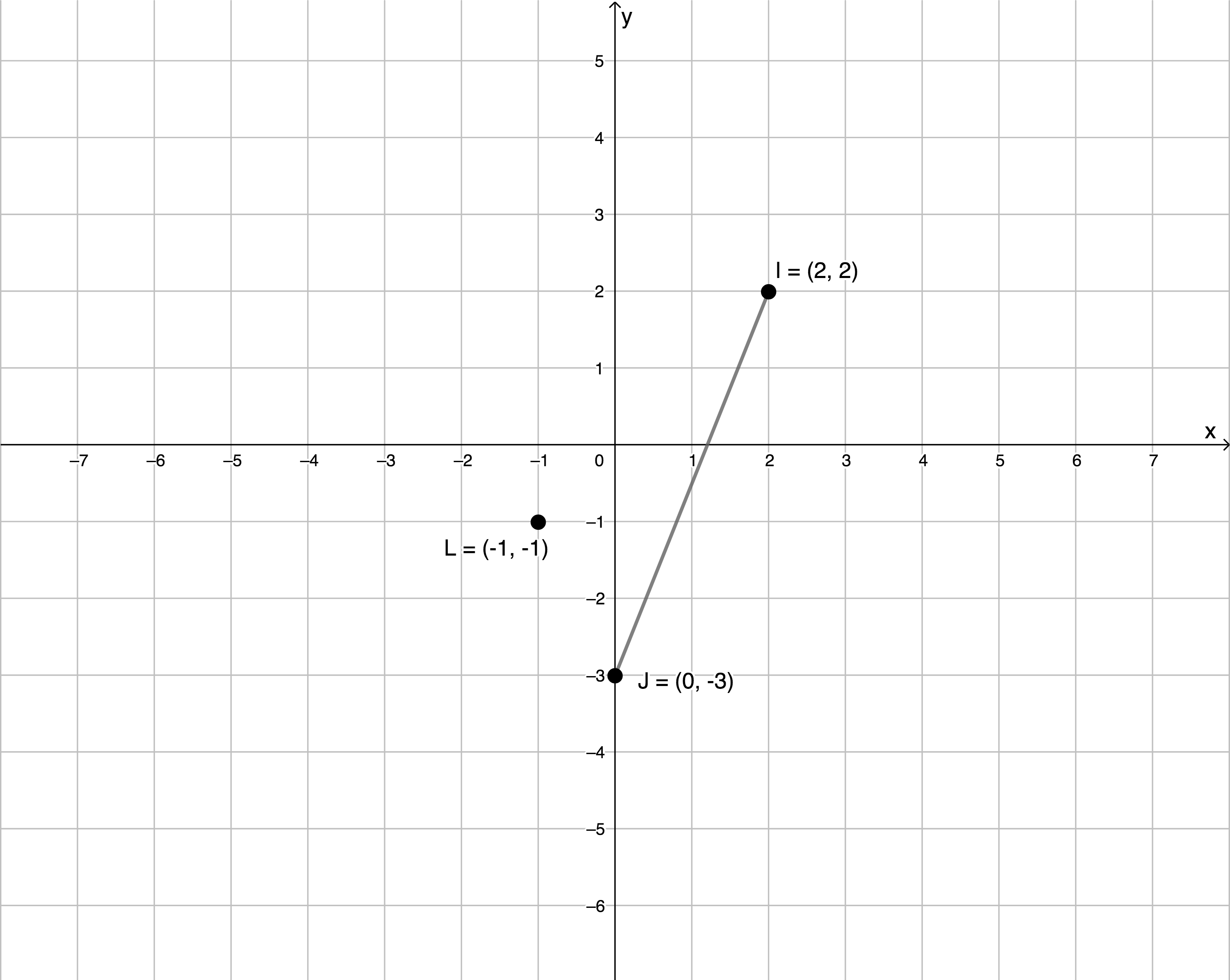We can see that to get from $\scriptsize \text{I}$ to $\scriptsize \text{J}$ we need to move two units to the left and then five units down. Therefore, if $\scriptsize \text{KL}$ is parallel to $\scriptsize \text{IJ}$ and the same length, the same movements will be necessary to get from one point to the other.

If we start at $\scriptsize \text{L}$ and move two units left and then five units down, we get to $\scriptsize (-3,-6)$. If we start at $\scriptsize \text{K}$ it will need to be the point $\scriptsize (1,4)$ so that when we move two units to the left and five units down we get to $\scriptsize \text{L}$. So, $\scriptsize \text{K}(1,4)$ or $\scriptsize \text{K}(-3,-6)$.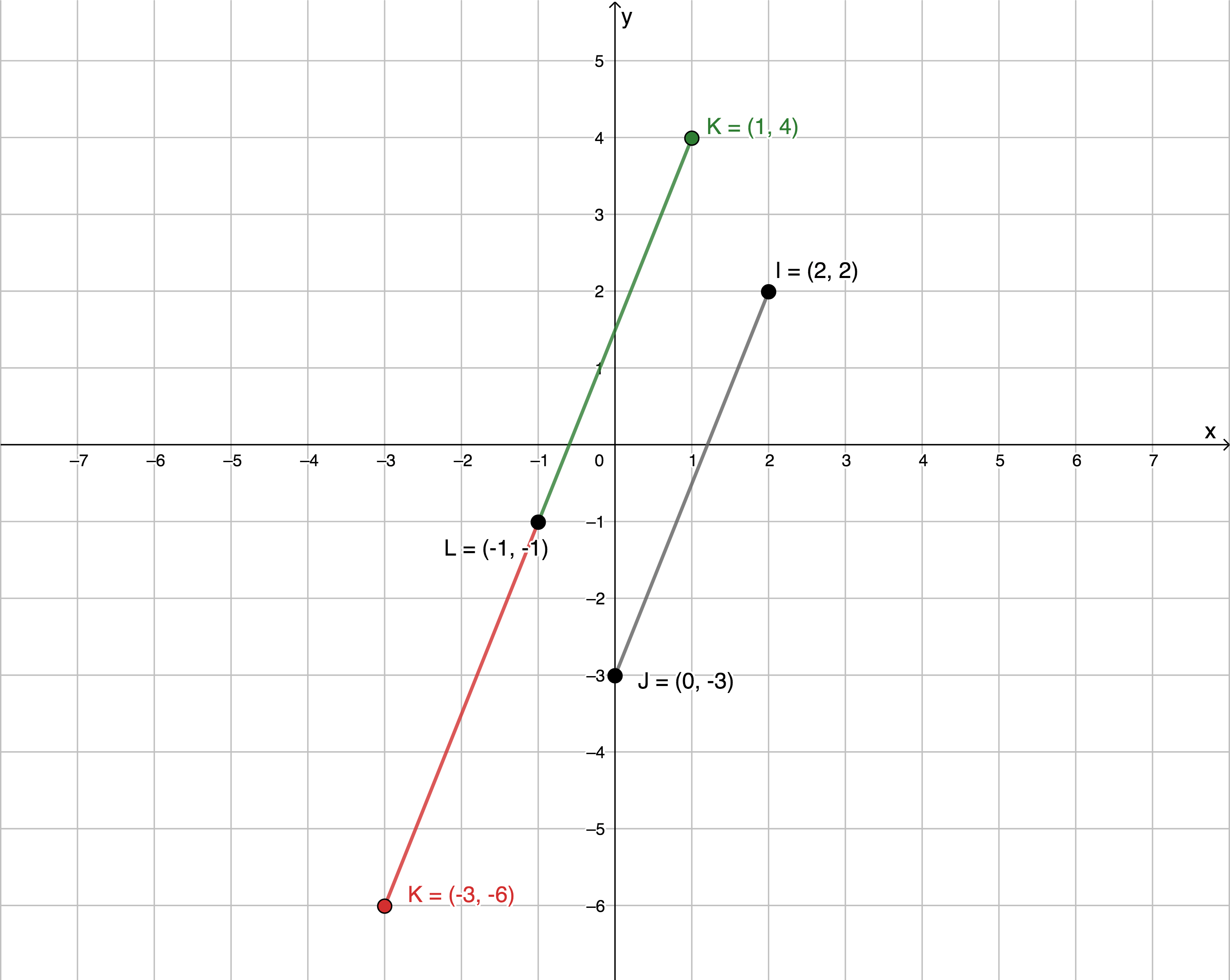Back to Unit 1: Assessment